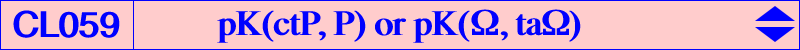Let pK(Ω, P) be the pivotal cubic with pole Ω = p:q:r and pivot P = u:v:w. The polar conics of A, B, C in this pK are centered at Oa, Ob, Oc. In general, the triangles ABC and OaObOc are not perspective unless Ω lies on the pivotal cubic with pole P^2 x ctP and pivot G/P where P^2 is the barycentric square of P, ctP is the complement of the isotomic conjugate of P (and also the center of the inconic with perspector P), G/P is the G–Ceva conjugate of P (and also the center of the circumconic with perspector P). Here x denotes the barycentric product. The most interesting case is obtained when Ω = ctP = P x cP since the triangles ABC and OaObOc are triply perspective. Any cubic pK(ctP, P) is a member of the class CL059. For example, with P = H we have Ω = K giving the Orthocubic K006. The coordinates of these points are : Oa = u(u+v)(u+w) : v(u+w)^2 : w(u+v)^2, Ob = u(v+w)^2 : v(v+u)(v+w) : w(v+u)^2, Oc = u(w+v)^2 : v(w+u)^2 : w(w+u)(w+v). The perspectors of ABC and OaObOc are : P0 = u(v+w)^2 : v(w+u)^2 : w(u+v)^2 = cP x ctP, P1 = u / (u+w) : v / (v+u) : w / (w+v), P2 = u / (u+v) : v / (v+w) : w / (w+u), to compare with P x tcP = u / (v+w) : v / (w+u) : w / (u+v). *** Construction and properties of these points Let Qa be the intersection of the line A cP and the parallel at tcP to BC. Qb and Qc are defined similarly. Let Q1 be the common point of the lines A tQc, B tQa, C tQb and Q2 the common point of the lines A tQb, B tQc, C tQa. P1 and P2 are the barycentric products Q1 x P and Q2 x P respectively. It follows that Oa = A P0 /\ B P2 /\ C P1, Ob = A P1 /\ B P0 /\ C P2 and Oc = A P2 /\ B P1 /\ C P0. Let Ea, Eb, Ec be the related points defined as follows : Ea = B Oc /\ C Ob = u(v+w) : v(u+v) : w(u+w), Eb = C Oa / A Oc = u(u+v) : v(u+w) : w(v+w), Ec = A Ob /\ B Oa = u(u+w) : v(v+w) : w(u+v). These three points are clearly related to ctP = P x cP = u(v+w) : v(w+u) : w(u+v) and they are the P x ctP–isoconjugates of Oa, Ob, Oc respectively. This same isoconjugation swaps P1 and P2, G and P x ctP, P0 and P x tcP. Note that the triangles OaObOc and EaEbEc are perspective at a point Q with barycentric coordinates u(u^2+v^2+w^2+uv+uw+3vw) : v(u^2+v^2+w^2+vu+vw+3uw) :w(u^2+v^2+w^2+wu+wv+3uv), showing that Q also lies on the line GP and on the line passing through ctP x cP and P x tcP. We shall meet this point again in the sequel. Furthermore, the two following sets of points lie on a same conic : Oa, Ob, Oc, P, P1, P2, ctP/P and Ea, Eb, Ec, P1, P2, ctP, P x ctP. *** Cubics related to triangles perspective to OaObOc Let Y be the G–Hirst conjugate of cP i.e. the intersection of the line through G, P, cP with the polar line of cP in the Steiner circum-ellipse. We have : Y = -u(u+v+w)+v^2+w^2+vw : : . Theorem 1 : OaObOc is perspective with the cevian triangle of any point on the cubic pKc with pole : cP x ctP^2 x Y, with pivot : ctP ÷Y (barycentric quotient), with isopivot : cP x ctP. Theorem 2 : OaObOc is perspective with the anticevian triangle of any point on the cubic pKa with pole : P x ctP, with pivot : P x Y, with isopivot : ctP ÷ Y. Theorem 3 : in both cases, the locus of the perspector is the cubic pKp with pole : P x ctP, with pivot : Q defined above, with isopivot : P x ctP ÷ Q. Theorem 4 : pKc and pKa generate a pencil of cubics which contains a third pivotal cubic pK3 with pole : ctP^2 x Y, with pivot : cP x ctP, with isopivot : P x Y.   The following table gives a selection of points that lie on these cubics. pK is pK(ctP, P). Recall that x and ÷ denote the barycentric product and quotient and that / denotes the cevian quotient. ccP is the complement of the complement of P and Z is the G–Hirst conjugate of P.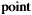1st barycentric coordinate pKc pKa pK3 pKp pK1 x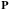u x xv+w x x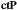u(v+w) x x xu(v+w)^2 x x x x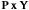u[-u(u+v+w)+v^2+w^2+vw] x x x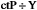u(v+w)÷[-u(u+v+w)+v^2+w^2+vw] x x x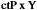u(v+w)[-u(u+v+w)+v^2+w^2+vw] x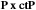u^2(v+w) xu(-u^2+v^2+w^2+uv+vw+wu) x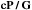-u^2+v^2+w^2+uv+vw+wu x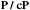u(v+w)(uv+uw+v^2+w^2)-vw(v+w)^2 x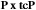u÷(v+w) x x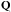u(u^2+v^2+w^2+uv+uw+3vw) x x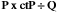u(v+w)÷(u^2+v^2+w^2+uv+uw+3vw) x x ctP x ccP u(v+w)(2u+v+w) x P ÷ ccP u÷(2u+v+w) x crossconjugate(P, ctP) u(v+w)÷(-u^2+v^2+w^2+uv+vw+wu) x ctP ÷ ccP u(v+w)÷(2u+v+w) x cP x ccP x ctP u(v+w)^2(2u+v+w) x ctP x Z u(v+w)(u^2-vw) x cP x ctP x Z u(v+w)^2(u^2-vw) x u(v+w)(2u^3+u^2v-uv^2-v^3+u^2w-uw^2-w^3) x ctP x ccP x Y u(v+w)(2u+v+w)[-u(u+v+w)+v^2+w^2+vw] x ctP ÷ ( ccP x Y) u(v+w)÷[(2u+v+w)[-u(u+v+w)+v^2+w^2+vw]] x P ÷ Z u÷(u^2-vw) x u(v+w)÷(2u^3+u^2v-uv^2-v^3+u^2w-uw^2-w^3) xRemarks • pKc, pKa, pK3 have already seven known common points namely A, B, C, ctP, cP x ctP, P x Y, ctP÷Y (yellow points in the table). The remaining two common points are imaginary. They are the intersections of the trilinear polar of ctP and the circumconic with perspector ctP. • the third points on the trilinear polar of ctP are the pink points in the table. • the sixth points on the circumconic with perspector ctP are the blue points in the table.